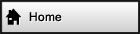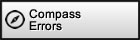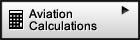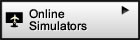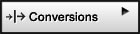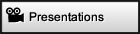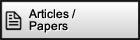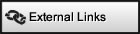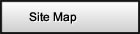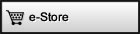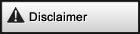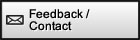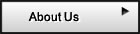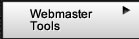Surf with confidence!   Site has been tested by:Norton™ Safe WebMcAfee SiteAdvisor ®Altimetry Help    True Altitude Your source for: Online Aviation Instrument Simulators + E6b, CR3 and other Flight Computer CalculatorsBack to calculator | 1 2 3 4 Next
 Using the True Altitude Calculator (1st calculator) Example 1: You are flying at 8000 ft indicated (or calibrated), your temperature instrument reads 4.6 oC for true air temperature (see note), and the altitude of the airport you obtained your altimeter setting is 1230 ft. Additionally, while maintaining altitude you momentarily set your altimeter to 29.92 inHg to find that the pressure altitude is 7620 ft, then you set it back to the setting it was before and continue to fly at 8000 ft indicated (or calibrated). Your true airspeed (TAS) is 220 kts. What is the true altitude that your aircraft is at? First let's list the information we have:      1) CA = 8000 ft      2) T = 4.6 oC      3) Station Altitude* = 1230 ft      4) PA = 7620 ft      5) TAS = 220 kts (not needed, unless we have total air temperature (TAT)            instead of true air temperature (T) - see note) * If you didn't have the station altitude, leave it at zero. The result will be not be as precise but you can still estimate the true airspeed using the calculator. Many flight computers ignore this also. We have four of the five variables for the calculator and therefore we have enough information to solve the problem using our first calculator. We will now enter the variables we have: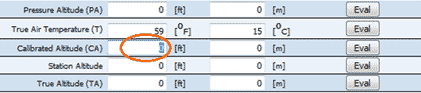Begin by highlighting the field that corresponds to calibrated altitude in ft.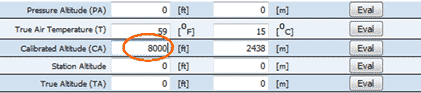Enter the 8000 value.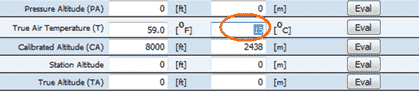Now highlight the field that corresponds to the true air temperature in oC.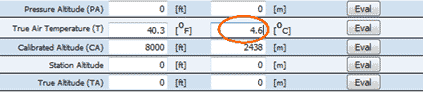Enter the 4.6 value.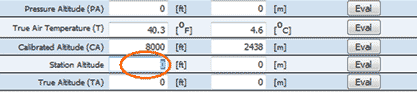Highlight the field that corresponds to the station altitude in ft.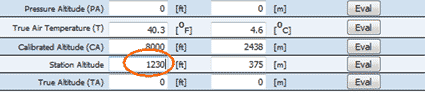Enter the 1230 value.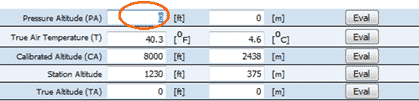Next highlight the field that corresponds to the pressure altitude in ft.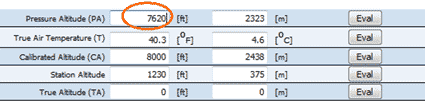Enter the 7620 value.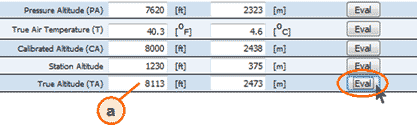The remaining field is the true altitude one. Press "Eval" and read the answer 8113 ft on (a). This is the best approximation for the actual or true altitude based on our data. Note Regarding True Air Temperature (T) Most general aviation aircraft have instruments that indicate total air temperature (TAT) also referred to as indicated air temperature (IAT). TAT is normally higher than true air temperature (T), also known as outside air temperature (OAT), because as the aircraft pushes the air, the air gets compressed. Compressed air increases in temperature. The temperature sensor therefore senses a slightly higher temperature than the actual outside air temperature. Some instruments in more sophisticated aircraft automatically make a correction for this. For slow moving aircraft the difference is minimal. For example, for aircraft that cruise at 100 knots, the difference is about 1.3 oC. In practice, in those slower aircraft, it is customary to use that total air temperature (TAT) in place of true air temperature (T) in calculations. In the example above, for simplification, we assumed we had the true air temperature (T). However if we did not have it, here is how we would calculate it: Assuming that we are at 220 knots (true airspeed) and our temperature instrument is measuring a total air temperature (TAT) of 11 oC, we can calculate true air temperature (T) using the following formula:      T = TAT - ΔT Remember T is the same thing as OAT; TAT is the same thing as IAT ΔT is the temperature rise, and can be found using the temperature rise calculator as demonstrated in the example below: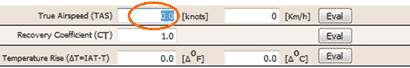In the temperature rise calculator, begin by highlighting the field that corresponds to true airspeed in knots.Enter the 220 value. Don't worry about the recovery coefficient. Just leave it at 1.0 unless you specifically know the value for your aircraft's temperature sensor.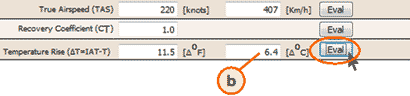The remaining field is the temperature rise one. Press "Eval" and read the answer 6.4 oC on (b). So in our case, if our temperature instrument is measuring total air temperature (TAT) of 11 oC , we have: 1) TAT = 11 oC (a given, not a calculated value) 2) ΔT = 6.4 oC (we just calculated it) It follows:      T = TAT - ΔT therefore:      T = 11 oC - 6.4 oC = 4.6 oC We would then have the true air temperature (T) to use in the true altitude calculator.
 Back to calculator | 1 2 3 4 Next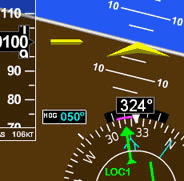Navigation Simulator Practice in our simulator specifically designed for learning the basics of instrument navigation. Try the Navigation Simulator, available for Windows. Free to try fully functional version for 10 days, \$39.95 to buy. Note: this simulator has its own self-contained Flash so it works without Flash being installed and is not subject to the Adobe Flash discontnuation / end of life.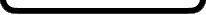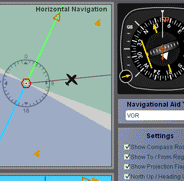Free Online Simulators No installation required. These simulators are ready to run on your web browser and have a rich set of features. Practice basic VOR, ADF, RMI and HSI intrument orientation and execute holding patterns. Other simulators include pitot static system and altimeter errors. Click here to go to main online simulator page.All rights reserved to Luiz Roberto Monteiro de Oliveira.  No content or code of this web site may be reproduced without prior permission from the author.
LuizMonteiro LLC d/b/a luizmonteiro.com